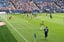# Image Similarity Search

Using Deep Lake for image similarity search

## How to Use Deep Lake as a Vector Store for Images

Deep Lake is a unique Vector Store because it supports storage of various data types including images, video, and audio. In this tutorial we show how to use Deep Lake to perform similarity search for images.

### Creating the Vector Store

We will use ~5k images in the COCO Validation Dataset as a source of diverse images. First, let's download the data.
We must unzip the images and specify their parent folder below.
Next, let's define a ResNet18 PyTorch model to embed the images based on the output from the second-to-last layer. We use the `torchvision` feature extractor to return the output of the `avgpool` layer to the `embedding` key, and we run on a GPU if available. (Note: `DeepLakeVectorStore` class was deprecated, but you can still use it. The new API for calling Deep Lake's Vector Store is: `VectorStore.`)
from deeplake.core.vectorstore.deeplake_vectorstore import VectorStore
import os
import torch
from torchvision import transforms, models
from torchvision.models.feature_extraction import create_feature_extractor
from PIL import Image
device = torch.device('cuda') if torch.cuda.is_available() else torch.device('cpu')
model = models.resnet18(pretrained=True)
return_nodes = {
'avgpool': 'embedding'
}
model = create_feature_extractor(model, return_nodes=return_nodes)
model.eval()
model.to(device)
Let's define an embedding function that will embed a list of image filenames and return a list of embeddings. A transformation must be applied to the images so they can be fed into the model, including handling of grayscale images.
tform= transforms.Compose([
transforms.Resize((224,224)),
transforms.ToTensor(),
transforms.Lambda(lambda x: torch.cat([x, x, x], dim=0) if x.shape == 1 else x),
transforms.Normalize([0.485, 0.456, 0.406], [0.229, 0.224, 0.225]),
])
def embedding_function(images, model = model, transform = tform, batch_size = 4):
"""Creates a list of embeddings based on a list of image filenames. Images are processed in batches."""
if isinstance(images, str):
images = [images]
#Proceess the embeddings in batches, but return everything as a single list
embeddings = []
for i in range(0, len(images), batch_size):
batch = torch.stack([transform(Image.open(item)) for item in images[i:i+batch_size]])
batch = batch.to(device)
embeddings+= model(batch)['embedding'][:,:,0,0].cpu().numpy().tolist()
return embeddings
Now we can create the vector store for storing the data. The Vector Store does not have the default configuration with `text`, `embedding`, and `metadata` tensors, so we use the `tensor_params` input to define the structure of the Vector Store.
vector_store_path = 'hub://<org_id>/<dataset_name>'
vector_store = VectorStore(
path = vector_store_path,
tensor_params = [{'name': 'image', 'htype': 'image', 'sample_compression': 'jpg'},
{'name': 'embedding', 'htype': 'embedding'},
{'name': 'filename', 'htype': 'text'}],
)
Finally, we can create a list of images from the source data and add it to the vector store.
image_fns = [os.path.join(images_path, item) for item in os.listdir(images_path) if os.path.splitext(item)[-1]=='.jpg']
filename = image_fns,
embedding_function = embedding_function,
embedding_data = image_fns)
We observe in the automatically printed summary that the Vector Store has tensors for the `image`, their `filename`, their `embedding`, and an `id`, with 5000 samples each. This summary is also available via `vector_store.summary()`.
tensor htype shape dtype compression
------- ------- ------- ------- -------
embedding embedding (5000, 512) float32 None
filename text (5000, 1) str None
id text (5000, 1) str None
image image (5000, 145:640, 200:640, 1:3) uint8 jpeg
Let's perform a similarity search on a reference image to find similar images in our Vector Store. First we download the image:image_similarity.jpg
112KB
Image
The similarity search will return data for the top k (defaults to 4) similar samples, including numpy arrays for the underlying images.
image_path = '/image_similarity.jpg'
result = vector_store.search(embedding_data = image_path,
embedding_function = embedding_function)
The key-value pairs in the result contains the tensor as the key and a list of values for the data:
result.keys()
# Returns: dict_keys(['filename', 'id', 'image', 'score'])
len(result['score'])
# Returns: 4
result['image'].shape
# Returns: (427, 640, 3)
Since images can be quite large, and we may not want to return them as numpy arrays, so we use `return_tensors` to specify that only the `filename` and `id` tensors should be returned:
result = vector_store.search(embedding_data = image_path,
embedding_function = embedding_function,
return_tensors = ['id', 'filename'])
result.keys()
# Returns: dict_keys(['filename', 'id', 'image', 'score'])

### Visualizing the Similarity Results

Instead of returning the results of the similarity search directly, we can use `return_view = True` to get the Deep Lake dataset view, which is a lazy pointer to the underlying data that satisfies the similarity search (no data is retrieved locally).
view = vector_store.search(embedding_data = image_path,
embedding_function = embedding_function,
return_view = True)
We can then save the view and visualize it in the Deep Lake UI:
view.save_view()The images are all fairly similar to the reference image, so it looks like the similarity search worked well!
Congrats! You just used the Deep Lake VectorStore in for image similarity search! 🎉